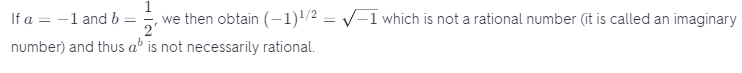# Prove Or Disprove That If A And B Are Rational Numbers, Then AB Is Also Rational.

We thoroughly check each answer to a question to provide you with the most correct answers. Found a mistake? Let us know about it through the REPORT button at the bottom of the page.

Prove or disprove that if a and b are rational numbers, then a^b is also rational.

The statement is false, one counterexample is for a = -1 and b = 1/2.

Given statement: If a and b are rational numbers, then a^b is also rational.

The given statement is false, which will be disproven using a counterexample.

Counterexample: